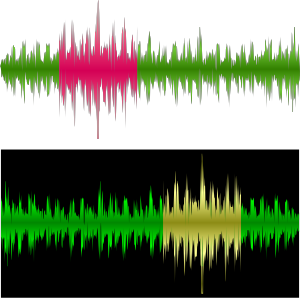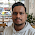## Saturday, 17 January 2015

### CBSE Class 9 - CH 12 - Sound (Study Points)

SoundVisual Representation of Sound Waveform (image courtsey: openclipart)

Study Points

1. Sound travels from one point to another in the form of waves.

2. Sound is produced due to vibration of different objects.

3. Sound waves are longitudinal in nature.

4. Sound travels as a longitudinal wave through a material medium.

5. Sound travels as successive compressions and rarefactions in the medium.

6. In sound propagation, it is the energy of the sound that travels and not the particles of the medium.

7. Sound cannot travel in vacuum.

8. Sound waves cannot be polarised.

9. The change in density from one maximum value to the minimum value and again to the maximum value makes one complete oscillation.

10. The part or region of a longitudinal wave in which the density of the particles of the medium is higher than the normal density is known as compression.

11. The part or region of a longitudinal wave in which the density of the particles of the medium is lesser than the normal density is called a rarefaction.

12. The distance between two consecutive compressions or two consecutive rarefactions is called the wavelength, λ.

13. The point of maximum positive displacement on a transverse wave is known as crest.

14. The point of maximum negative displacement on a transverse wave is known as through.

15. The time taken by the wave for one complete oscillation of the density or pressure of the medium is called the time period, T.

16. The distance traveled by a wave in one second is called wave velocity. It depends upon the nature of the medium through which it passes.

17. A wave or short duration which is confined to a small portion of a medium at any given time is known as a pulse.

18. The number of complete oscillations per unit time is called the frequency (ν), ν = 1/T .

19. Unit of frequency is Hertz (Hz).

20. The speed v, frequency ν, and wavelength λ, of sound are related by the equation, v = λ/ν.

21. The speed of sound depends primarily on the nature and the temperature of the transmitting medium.

22. There is no effect of pressure on the speed of sound if the temperature is kept constant.

23. Speed of sound increases with the increase in sound temperature.

24. Speed of sound increases with the increase in humidity of air.

25. Sound travels faster in solids than in air. The speed of sound in solids is much more than the speed of sound in liquids or gases.

26. Bells are made of metals because of high elasticity of metals. (Sound propagates in elastic medium).

27. The speed of sound is higher in rainy season than in summers.

28. Sound exhibits reflection, resonance, interference etc.

29. The law of reflection of sound states that the directions in which the sound is incident and reflected make equal angles with the normal to the reflecting surface and the three lie in the same plane.

30. For hearing a distinct sound, the time interval between the original sound and the reflected one must be at least 0.1 s.

31. Echo is a repetition of sound due to the reflection of original sound by a large and hard obstacle.

32. Echo is produced by the reflection of sound.

33. The persistence of sound in an auditorium is the result of repeated reflections of sound and is called reverberation.

34. Sound properties such as pitch, loudness and quality are determined by the corresponding wave properties.

35. Loudness is a physiological response of the ear to the intensity of sound.

36. The amount of sound energy passing each second through unit area is called the intensity of sound.

37. Decibel (dB) is the unit to measure sound loudness/intensity or sound pressure levels.

38. A normal talk between two persons is 60 dB. Any sound of intensity equal to or greater than 85dB can damage human hearing.

39. The audible range of hearing for average human beings is in the frequency range of 20 Hz – 20 kHz.

40. Sound waves with frequencies below the audible range are termed “infrasonic” and those above the audible range are termed “ultrasonic”.

41. Ultrasound has many medical and industrial applications.

42. The SONAR technique is used to determine the depth of the sea and to locate under water hills, valleys, submarines, icebergs, sunken ships etc.1.how to copy paste these things man...?

1.2.burrrrrnnn

3.with allow copy chrome extennsion

2.What is point 20?